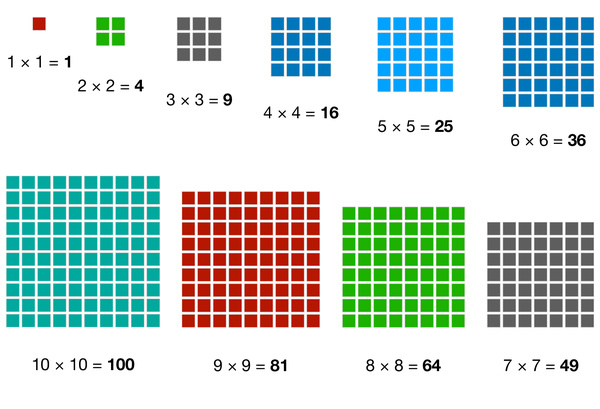# Number Blocks: First Ten Square NumbersSquare numbers are numbers that result from multiplying a number by itself. For example, 3 x 3 = 9, so 9 is a square number. Here are the first ten square numbers:

1 (1 x 1 = 1)
4 (2 x 2 = 4)
9 (3 x 3 = 9)
16 (4 x 4 = 16)
25 (5 x 5 = 25)
36 (6 x 6 = 36)
49 (7 x 7 = 49)
64 (8 x 8 = 64)
81 (9 x 9 = 81)
100 (10 x 10 = 100)

Understanding square numbers is an important concept in math, as it helps build a foundation for more advanced topics like algebra and geometry. Remembering the first ten square numbers is a great way to start building a strong foundation in math!

The term "square" comes from the fact that if you draw a square with sides of length n units, the area of the square is n^2 units. For example, if you draw a square with sides of length 3 units, the area of the square is 3^2 = 9 square units.

Square numbers have some interesting patterns. For example, if you take the difference between consecutive square numbers, you get a sequence of odd numbers. For instance, the difference between 1 and 4 is 3, the difference between 4 and 9 is 5, and so on.

Square numbers are used in many real-world applications, such as calculating the area of a square or rectangular object, calculating the distance between two points on a coordinate plane, and even in cryptography to encrypt messages.

In addition to the first ten square numbers listed above, here are a few more: 121, 144, 169, 196, 225, 256, 289, 324, 361.

By understanding and memorizing the first ten square numbers and their properties, kids can build a solid foundation in math that will serve them well throughout their academic careers.